# Using natural splines in linear modeling

Take a look at this scatterplot: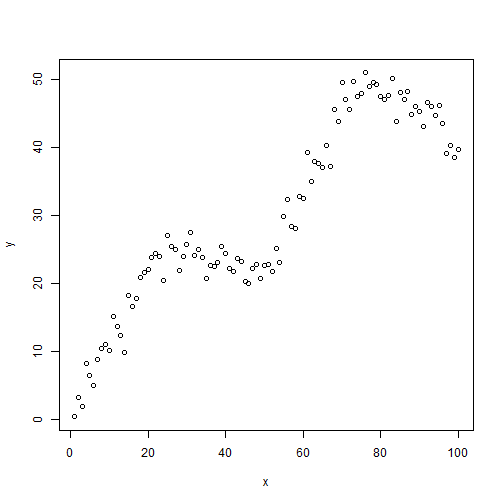It's clear there is a relationship between x and y, but the relationship is non-linear. How can we fit a linear model to this data? We could try fitting a polynomial model. The relationship seems to “change directions” four different times, so we could try fitting a 4th-degree polynomial model.

modp <- lm(y ~ poly(x, 4))
plot(x,y)
lines(x, fitted(modp))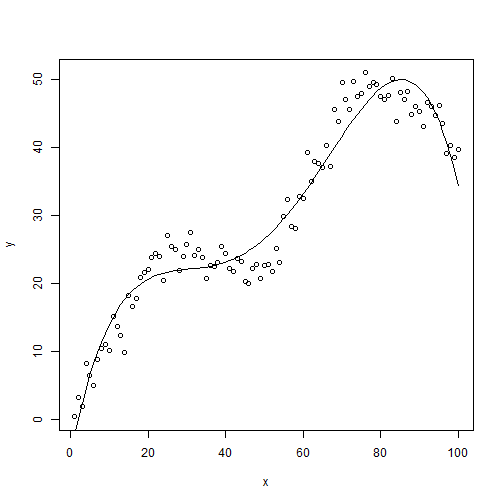This sort of captures the general nature of the relationship but the peaks and valleys just aren't quite right. They either over-predict or under-predict. We would like to do better.

Another approach might be to use a nonparametric regression approach such as loess. If we set the span parameter to 0.5, which controls the amount of smoothing, we get a decent fitting model:

modl <- loess(y ~ x, span = 0.5)
plot(x, y)
lines(x, predict(modl))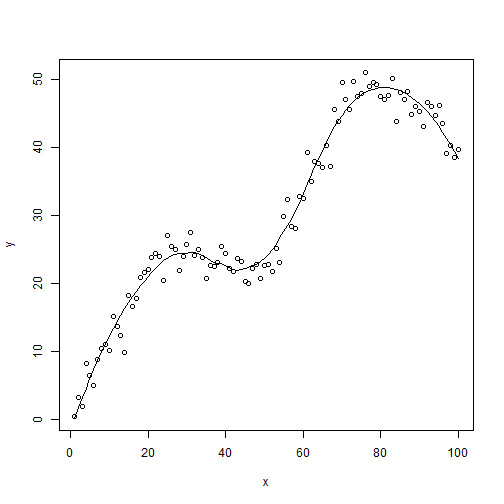This matches what we get when we use ggplot with the smooth geom:

library(ggplot2)
ggplot(data.frame(x, y), aes(x, y)) +
geom_point() +
geom_smooth(se = F, span = 0.5)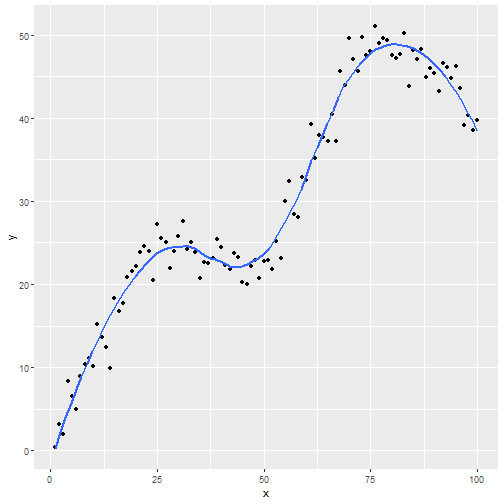But the drawback is we have no prediction equation. This is a non-parametric approach, hence no parameters were estimated.

This leads us to restricted cubic splines, or natural splines. The basic idea is to model a non-linear relationship such as the one in our example with piecewise cubic polynomials. Let's go ahead and first use natural splines in our linear model and then talk a little more about what's happening behind the scenes. Below we first load the splines package (a recommended package that comes with base R) so we have access to the ns function (natural splines). Notice we call ns on our predictor and specify df as 4. Specifying df = 4 implies 3 interior knots (ie, not including two “boundary knots”).

library(splines)
modns <- lm(y ~ ns(x, df = 4))
plot(x, y)
lines(x, predict(modns))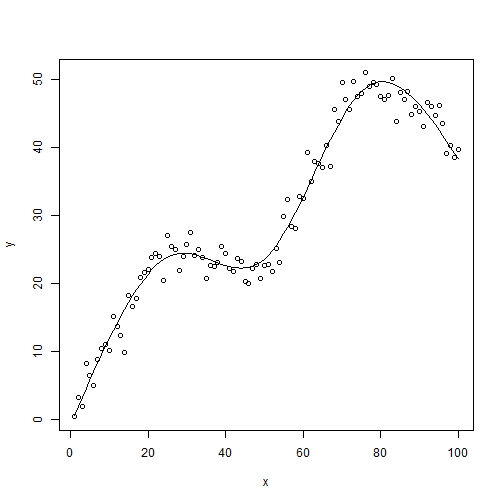This looks better than the polynomial model. And unlike the loess fit, we have a prediction equation:

summary(modns)

##
## Call:
## lm(formula = y ~ ns(x, df = 4))
##
## Residuals:
##    Min     1Q Median     3Q    Max
## -6.355 -1.302 -0.052  1.279  5.325
##
## Coefficients:
##                Estimate Std. Error t value Pr(>|t|)
## (Intercept)      0.6489     0.8242   0.787    0.433
## ns(x, df = 4)1  11.9996     1.0508  11.420   <2e-16 ***
## ns(x, df = 4)2  50.1753     1.0438  48.071   <2e-16 ***
## ns(x, df = 4)3  72.0807     2.1079  34.195   <2e-16 ***
## ns(x, df = 4)4  19.5918     0.9794  20.004   <2e-16 ***
## ---
## Signif. codes:  0 '***' 0.001 '**' 0.01 '*' 0.05 '.' 0.1 ' ' 1
##
## Residual standard error: 2.114 on 95 degrees of freedom
## Multiple R-squared:  0.977,  Adjusted R-squared:  0.976
## F-statistic:  1008 on 4 and 95 DF,  p-value: < 2.2e-16


Obviously the coefficients defy interpretation, but we can work with them as we would any other linear model. For example, we can test for linearity, that is $$H_0: \beta_2 = \beta_3 = \beta_4 = 0$$. In our model that means testing that the last 3 coefficients are equal to 0. The car package provides the powerful linearHypothesis function for this purpose.

library(car)
linearHypothesis(modns, names(coef(modns))[3:5])

## Linear hypothesis test
##
## Hypothesis:
## ns(x, df = 4)2 = 0
## ns(x, df = 4)3 = 0
## ns(x, df = 4)4 = 0
##
## Model 1: restricted model
## Model 2: y ~ ns(x, df = 4)
##
##   Res.Df     RSS Df Sum of Sq      F    Pr(>F)
## 1     98 18311.8
## 2     95   424.4  3     17887 1334.7 < 2.2e-16 ***
## ---
## Signif. codes:  0 '***' 0.001 '**' 0.01 '*' 0.05 '.' 0.1 ' ' 1


It's no surprise that the test is highly significant. We can also verify our model with natural splines is superior to the polynomial model via AIC. (Recall a lower AIC is better.)

AIC(modp, modns)

##       df      AIC
## modp   6 521.2002
## modns  6 440.3375


Now that we have played with natural splines, let's back up and try to get a better understanding of what's going on. To begin with, here's how we generated the data:

x <- 1:100         # independent variable
k <- c(25, 50, 75) # 3 interior knots

# function to construct variables x2, x3, x4
u <- function(x)ifelse(x > 0, x, 0)

x2 <- u(x - k)
x3 <- u(x - k)
x4 <- u(x - k)

# generate data
set.seed(1)
y <- 0.8 + 1*x + -1.2*x2 + 1.4*x3 + -1.6*x4 + rnorm(100,sd = 2.2)
plot(x, y)Our first predictor is x, which is simply the numbers 1 – 100. Next we define 3 “knots” at 25, 50, and 75. We then use those knots to construct three additional variables: x2, x3, and x4.

• x2 is equal to x – 25 when x – 25 is greater than 0
• x3 is equal to x – 50 when x – 50 is greater than 0
• x4 is equal to x – 75 when x – 75 is greater than 0

Finally we generate our dependent variable, y, as a function of x, x2, x3, and x4 plus some noise from a Normal(0, 2.2) distribution. The formula on the right side of the assignment operator is our True model. This is technically called a linear spline function. The best linear model we could fit to the data would be the following:

mod <- lm(y ~ x + x2 + x3 + x4)
summary(mod)

##
## Call:
## lm(formula = y ~ x + x2 + x3 + x4)
##
## Residuals:
##     Min      1Q  Median      3Q     Max
## -5.0699 -1.2797  0.1007  1.2322  4.9155
##
## Coefficients:
##             Estimate Std. Error t value Pr(>|t|)
## (Intercept)  1.34673    0.77485   1.738   0.0854 .
## x            0.97506    0.04425  22.035   <2e-16 ***
## x2          -1.14818    0.07094 -16.186   <2e-16 ***
## x3           1.35246    0.06220  21.743   <2e-16 ***
## x4          -1.57494    0.06865 -22.941   <2e-16 ***
## ---
## Signif. codes:  0 '***' 0.001 '**' 0.01 '*' 0.05 '.' 0.1 ' ' 1
##
## Residual standard error: 2.01 on 95 degrees of freedom
## Multiple R-squared:  0.9792, Adjusted R-squared:  0.9783
## F-statistic:  1117 on 4 and 95 DF,  p-value: < 2.2e-16

plot(x, y)
lines(x, fitted(mod))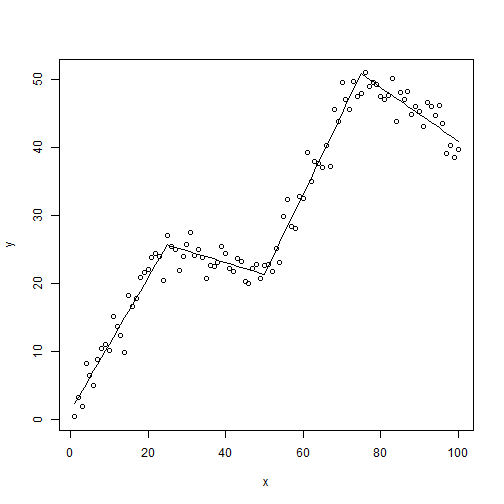So we see that to make the trajectory of our data change directions 4 times, we needed to create 4 predictors, 3 of which were based on one. This might make intuitive sense if we think of a simple parabola. It changes directions twice and has two coefficients for the $$x$$ and $$x^2$$ parameters. It's a 2nd degree polynomial. Likewise for a 3rd degree polynomial, a 4th degree polynomial, and so forth. There's only one $$x$$, but the trajectory of $$y$$ changes depending on the degree of the polynomial.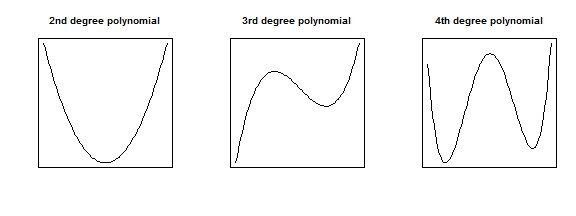The takeaway is that when we see that our response variable has a non-linear relationship with a predictor variable in real life, we may need to consider more than just a single slope coefficient to model the relationship. In the example we've been using the slope, or trajectory, changes directions 4 times, which suggests using four predictors instead of one. We tried a 4th degree polynomial of $$x$$ but saw that didn't work as well as we would have liked. A recommended approach then is to try natural splines.

The basic, and I mean very basic, idea of natural splines is to fit a 3rd degree polynomial to data within knots, and then connect those lines together. For example, below is our data with knots defined at 0, 25, 50, 75, and 100.

plot(x,y)
abline(v = c(0,25,50,75,100))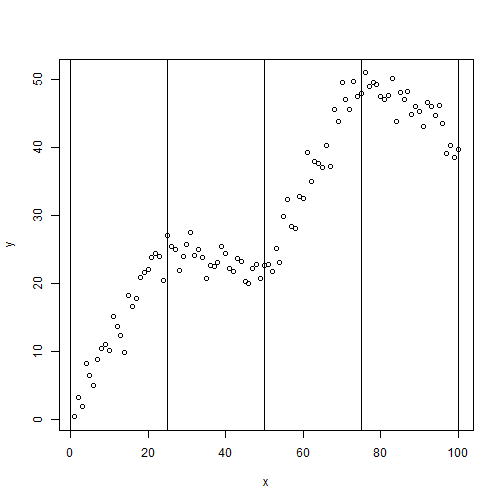With 5 knots, we have 4 regions of data. Within those 4 regions of data, natural splines essentially allow us to fit 4 different 3rd degree polynomials, all smoothed together. The magic is in how the 3 additional predictors are generated. Using the ns function in the splines package, we can create a basis matrix that allows us to fit a natural cubic spline using regular regression functions such as lm and glm. How the basis matrix is generated is quite complicated and probably something you'll just want to take on faith, like I do.

We can sort of see the natural spline in action if we fit and then color the lines between the knots. Below we regress $$y$$ on a natural spline of $$x$$ with knots defined at 25, 50 and 70. We then color the fitted lines differently between the knots.

plot(x,y)
mod.ns <- lm(y ~ ns(x, knots = c(25,50,75)))
lines(x[1:25], fitted(mod.ns)[1:25],col=1)
lines(x[26:50], fitted(mod.ns)[26:50],col=2)
lines(x[51:75], fitted(mod.ns)[51:75],col=3)
lines(x[76:100], fitted(mod.ns)[76:100],col=4)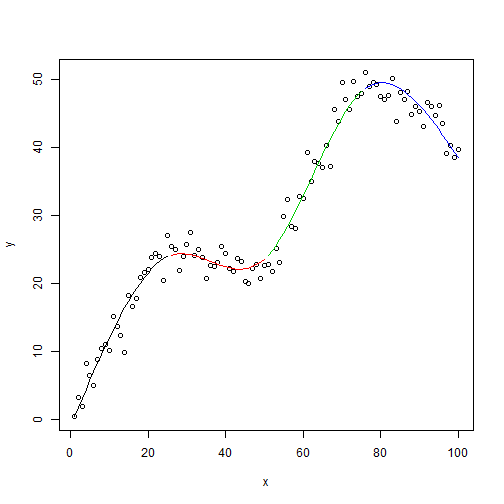Notice the red and green interior lines have a noticeable 3rd degree polynomial shape. The exterior red and blue lines look more like a 2nd degree polynomial. That's because natural splines are constrained to be linear in the tails (ie, the boundary knots). For this reason, natural splines are sometimes called restricted cubic splines. If we didn't want that constraint, we could use the bs function to generate a b-spline matrix basis. Here's what that looks like.

plot(x,y)
mod.bs <- lm(y ~ bs(x, knots = c(25,50,75)))
lines(x[1:25], fitted(mod.bs)[1:25],col=1)
lines(x[26:50], fitted(mod.bs)[26:50],col=2)
lines(x[51:75], fitted(mod.bs)[51:75],col=3)
lines(x[76:100], fitted(mod.bs)[76:100],col=4)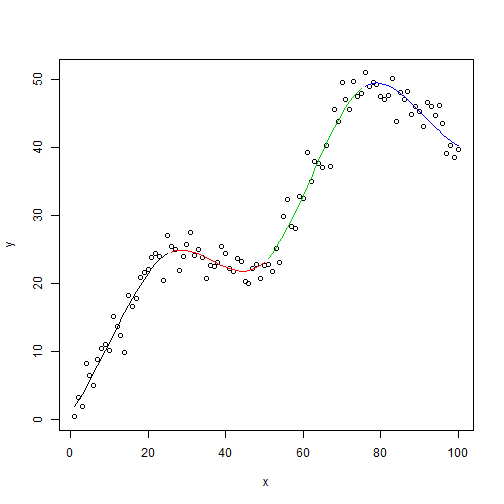Notice the blue line in particular curls up at the boundary as would be expected of a 3rd degree polynomial. But that's not the only difference. Look at the summary output. Notice the bs function generated matrix with 6 columns instead of 4.

summary(mod.bs)

##
## Call:
## lm(formula = y ~ bs(x, knots = c(25, 50, 75)))
##
## Residuals:
##     Min      1Q  Median      3Q     Max
## -5.8247 -1.1582 -0.0468  1.2780  5.0283
##
## Coefficients:
##                               Estimate Std. Error t value Pr(>|t|)
## (Intercept)                      1.983      1.218   1.628  0.10699
## bs(x, knots = c(25, 50, 75))1    6.623      2.281   2.903  0.00461 **
## bs(x, knots = c(25, 50, 75))2   33.328      1.529  21.794  < 2e-16 ***
## bs(x, knots = c(25, 50, 75))3    8.281      1.840   4.501 1.96e-05 ***
## bs(x, knots = c(25, 50, 75))4   60.284      1.722  35.000  < 2e-16 ***
## bs(x, knots = c(25, 50, 75))5   40.697      1.913  21.278  < 2e-16 ***
## bs(x, knots = c(25, 50, 75))6   38.377      1.688  22.736  < 2e-16 ***
## ---
## Signif. codes:  0 '***' 0.001 '**' 0.01 '*' 0.05 '.' 0.1 ' ' 1
##
## Residual standard error: 2.052 on 93 degrees of freedom
## Multiple R-squared:  0.9788, Adjusted R-squared:  0.9774
## F-statistic: 714.2 on 6 and 93 DF,  p-value: < 2.2e-16


So fitting a b-spline means actually fitting a more complicated model. For this reason and the fact that b-splines can be poory behaved in the tails (Harrell, p. 24), most statisticians recommend working with natural splines.

To wrap up, let's go over some guidelines for using natural splines with real data. First off, you don't want to go looking at your data and guessing how many times it changes direction to determine your knots or degrees of freedom! I did that simply as a way to help explain how splines worked. In practice you'll want to determine degrees of freedom based on sample size and how important you suspect a predictor to be. Harrell states that 4 degrees of freedom is usually sufficient. If your sample is on the small side, perhaps choose 3 degrees of freedom. If it's large, go with 5. And notice we're talking about degrees of freedom, not knots. The location of knots isn't that crucial and ns will automatically select knots based on the quantiles of the predcitor.

Something else to remember is that the coefficients on a model with natural splines defy any sort of interpretation. So forget using the “1-unit increase in x leads to a __ increase in y” method to explain association. An alternative approach is an effect plot, which allows you to visualize your model given certain predictor values. Here's a quick demonstration using a simplified example that comes with the powerful effects package. Below we model the log of prestige, a prestige score for someone's occupation, as a function of logged income and a 4 degree of freedom natural spline basis of education. Calling summary and anova on the model object will reveal the natural spline appears warranted and highly significant.

library(effects)
mod.pres1 <- lm(log(prestige) ~ log(income) + ns(education, 4),
data=Prestige)


But what does it mean? What is the association between prestige and education when, say, holding income at the mean value? An effect plot sheds some light.

eff.log <- Effect("education", mod.pres1, transformation=list(inverse=exp))
plot(eff.log)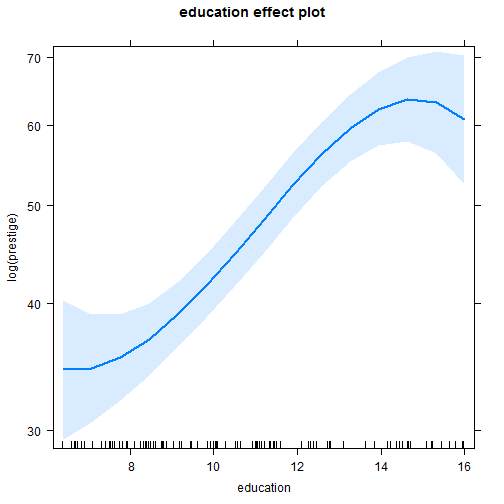This shows that from about 9 – 14, the effect of education is pretty dramatic on prestige scores, but rather uncertain in the extremes, below 9 and above 14. From 10 – 14, it looks like a 2-level increase in education is worth about a 10 point increase in prestige scores. We couldn't guess that from the summary output but we can sort of infer it from the effect plot. Again, this is just an example and not a replication of the original analysis.

Reference:

F. Harrell. Regression Modeling Strategies: With Applications to Linear Models, Logistic and Ordinal Regression, and Survival Analysis. 2nd Ed Springer. 2015

## 1 thought on “Using natural splines in linear modeling”

1. Roksolana Petrovska

I wonder, how to backtransform linear regression with splines and logged dependent variable? Suppose, I have the regression like:
log(Y) = ns(X1, 5)+ns(X2, 5)+X3(categorical)
and with the predict function I would like to back-transform Y. Usually, I exponentiate the fitted values and standard deviation as following:
exp((summary(fit)\$sigma)^2/2)*exp(predict(fit))
In case of splines, I am lost how to back-transform. GLM with log-link function is an option but it shows not a very good fit. Could you advise? Thank you in advance!

With the best wishes,
Roksolana

This site uses Akismet to reduce spam. Learn how your comment data is processed.